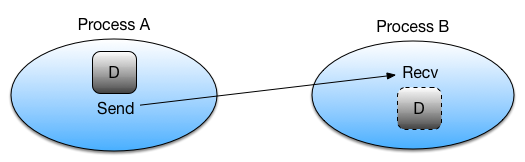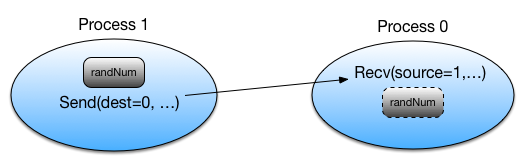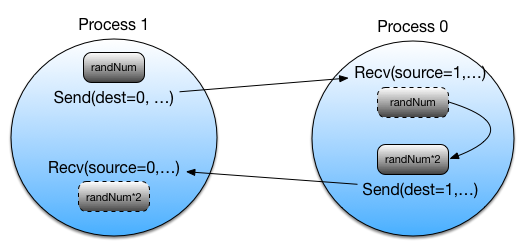Message Passing

Overview

Teaching: 40 min
Exercises: 20 min
Questions
• What are the fundamental communication primitives?

• What is point-to-point communication?

Objectives
• Write your first MPI program.

• Understand communication primitives.

The most commonly used method of programming distributed-memory MIMD systems is message passing, or some variant of message passing. MPI is the most widely used standard.

In basic message passing, the processes coordinate their activities by explicitly sending and receiving messages. Explicit sending and receiving messages is known as point to point communication.

MPI’s send and receive calls operate in the following manner:

• First, process A decides a message needs to be sent to process B.
• Process A then packs up all of its necessary data into a buffer for process B.
• Process A indicates that the data should be sent to process B by calling the Send function.
• Before process B can receive the data, it needs to acknowledge that it wants to receive it. Process B does this by calling the Recv function.

In this way, every time a process sends a message, there must be a process that also indicates it wants to receive the message. i.e. calls to Send and Recv are always paired.How does a process know where to send a message?

The number of processes is fixed when an MPI program is first started (there is a way to create more processes, but we will ignore that for now.) Each of the processes is assigned a unique integer starting from 0. This integer is know as the rank of the process and is how each process is identified when sending and receiving messages.

MPI processes are arranged in logical collections that define which processes are allowed to send and receive messages. A collection of this type is known as communicator. Communicators can be arranged in an hierarchy, but as this is seldom used in MPI, we will not consider it more here. There is one special communicator that exists when an MPI program starts, that contains all the processes in the MPI program. This communicator is called MPI.COMM_WORLD. In mpi4py, communicators are represented by the Comm class.

In order for a process to learn about other processes, MPI provides two methods on a communicator.

The first of these is called Get_size(), and this returns the total number of processes contained in the communicator (the size of the communicator).

The second of these is called Get_rank(), and this returns the rank of the calling process within the communicator. Note that Get_rank() will return a different value for every process in the MPI program.

Terminology

For simplicity, we will refer to the “process who’s rank is N” and “process N” as being the same process.

The following code obtains the size of the MPI.COMM_WORLD communicator, and rank of the process within the communicator. We will create a file called mpi1.py and run this code to see what the values of size and rank are for each process.

from mpi4py import MPI

comm = MPI.COMM_WORLD
size = comm.Get_size()
rank = comm.Get_rank()
print('size=%d, rank=%d' % (size, rank))

Now run the program using the command:

mpiexec -n 4 python mpi1.py

You should see output similar to the following:

size=4, rank=1
size=4, rank=0
size=4, rank=2
size=4, rank=3

What do you notice about the order that the program prints the values in? Hint: try running the program a few times and see what happens.

mpiexec

The mpiexec command is how an MPI program is started. The -n argument specifies how many processes (tasks) to start. It is possible to start an MPI with more or less processes than the program is expecting, so it is always a good idea to design the code so that it will accept any number of processes. Alternatively, check how many processes have been started and only run if the number is what you expect.

One MPI program, multiple MPI processes

When an MPI program is run, each process consists of the same code. However, as we’ve seen, there is one, and only one, difference: each process is assigned a different rank value. This allows code for each process to be embedded within one program file.

In the following code, all processes start with the same two numbers a and b. However, although there is only one file, each process performs a different computation on the numbers. Process 0 prints the sum of the numbers, process 1 prints the result of multiplying the numbers, and process 2 prints the maximum value.

Create a program called mpi2.py containing the code below.

from mpi4py import MPI
rank = MPI.COMM_WORLD.Get_rank()

a = 6.0
b = 3.0
if rank == 0:
print(a + b)
if rank == 1:
print(a * b)
if rank == 2:
print(max(a,b))

Run this program using the command:

mpiexec -n 3 python mpi2.py

You should now see output similar to:

9.0
18.0
6.0

Challenge

Modify the above program to add the statement print("end of MPI") as the last line. What happens when you run the program now? What happens if you increase the number of processes to 4? Can you explain what you are seeing?

Point-to-point communication

As mentioned in earlier, the simplest message passing involves two processes: a sender and a receiver. Let us begin by demonstrating a program designed for two processes. One will draw a random number and then send it to the other. We will do this using the routines Send and Recv.

Create a program called mpi3.py containing the code below.

import numpy
from mpi4py import MPI
comm = MPI.COMM_WORLD
rank = comm.Get_rank()

randNum = numpy.zeros(1)

if rank == 1:
randNum = numpy.random.random_sample(1)
print("Process", rank, "drew the number", randNum)
comm.Send(randNum, dest=0)

if rank == 0:
print("Process", rank, "before receiving has the number", randNum)
comm.Recv(randNum, source=1)
print("Process", rank, "received the number", randNum)

Run this program using the command:

mpiexec -n 2 python mpi3.py

This program generates the following output:

Process 0 before receiving has the number 0.0
Process 0 received the number 0.815583406506
Process 1 drew the number 0.815583406506

The Send and Recv functions are referred to as blocking functions (we will look at non-blocking functions later). If a process calls Recv it will simply wait until a message from the corresponding Send is received before proceeding. Similarly the Send will wait until the message has been reveived by the corresponding Recv.Because Send and Recv are blocking functions, a very common situation that can occur is called deadlock. This happens when one process is waiting for a message that is never sent. We can see a simple example of this by commenting out the comm.Send and running the program below.

import numpy
from mpi4py import MPI
comm = MPI.COMM_WORLD
rank = comm.Get_rank()

randNum = numpy.zeros(1)

if rank == 1:
randNum = numpy.random.random_sample(1)
print("Process", rank, "drew the number", randNum)
#comm.Send(randNum, dest=0)

if rank == 0:
print("Process", rank, "before receiving has the number", randNum)
comm.Recv(randNum, source=1)
print("Process", rank, "received the number", randNum)

What do you see when you run this code?

As the program is deadlocked, you will need kill the program using ^C (Control-C) in order to continue.

Notice how easy it is for the program to result in a deadlock! We only had to change one statement for this to happen.

More Send and Recv

Previously, we saw how to send a message from one process to another. Now we’re going to try sending a message to a process and receiving a message back again.

Let’s modify the previous code so that when the process 0 receives the number, it multiplies it by two and sends it back to process 1. Process 1 should then print out the new value.Create a new program called mpi4.py with the following code:

import numpy
from mpi4py import MPI
comm = MPI.COMM_WORLD
rank = comm.Get_rank()

randNum = numpy.zeros(1)

if rank == 1:
randNum = numpy.random.random_sample(1)
print("Process", rank, "drew the number", randNum)
comm.Send(randNum, dest=0)
comm.Recv(randNum, source=0)
print("Process", rank, "received the number", randNum)

if rank == 0:
print("Process", rank, "before receiving has the number", randNum)
comm.Recv(randNum, source=1)
print("Process", rank, "received the number", randNum)
randNum *= 2
comm.Send(randNum, dest=1)

Run this program using the command:

mpiexec -n 2 python mpi4.py

Here is the output you should see:

Process 0 before receiving has the number 0.0
Process 0 received the number 0.405456788104
Process 1 drew the number 0.405456788104
Process 1 received the number 0.810913576208

The receiving process does not always need to specify the source when issuing a Recv. Instead, the process can accept any message that is being sent by another process. This is done by setting the source to MPI.ANY_SOURCE.

We can try replacing the source=N arguments in your program with source=MPI.ANY_SOURCE to see if it still works.

import numpy
from mpi4py import MPI
comm = MPI.COMM_WORLD
rank = comm.Get_rank()

randNum = numpy.zeros(1)

if rank == 1:
randNum = numpy.random.random_sample(1)
print("Process", rank, "drew the number", randNum)
comm.Send(randNum, dest=0)
comm.Recv(randNum, source=MPI.ANY_SOURCE)
print("Process", rank, "received the number", randNum)

if rank == 0:
print("Process", rank, "before receiving has the number", randNum)
comm.Recv(randNum, source=MPI.ANY_SOURCE)
print("Process", rank, "received the number", randNum)
randNum *= 2
comm.Send(randNum, dest=1)

Now we should see output like the following:

Process 0 before receiving has the number 0.0
Process 0 received the number 0.94706618094
Process 1 drew the number 0.94706618094
Process 1 received the number 1.89413236188

A Final word on the Send and Recv methods

Here are the actual definitions of the Send and Recv methods:

Comm.Send(buf, dest=0, tag=0)

Performs a basic send. This send is a point-to-point communication. It sends information from exactly one process to exactly one other process.

Parameters:

• Comm (MPI comm) – communicator we wish to query
• buf (choice) – data to send
• dest (integer) – rank of destination
• tag (integer) – message tag

Comm.Recv(buf, source=0, tag=0, status=None)

Performs a point-to-point receive of data.

Parameters:

• Comm (MPI comm) – communicator we wish to query
• buf (choice) – initial address of receive buffer (choose receipt location)
• source (integer) – rank of source
• tag (integer) – message tag
• status (Status) - status of object

Sometimes there are cases when a process might have to send many different types of messages to another process. Instead of having to go through extra measures to differentiate all these messages, MPI allows senders and receivers to also specify message IDs (known as tags) with the message. The receiving process can then request a message with a certain tag number and messages with different tags will be buffered until the process requests them.

Key Points

• MPI uses the notion of a rank to distinguish processes.

• Send and Recv are the fundumental primitives.

• Sending a message from one process to another is known as point-to-point communication.